# 关于几个公式的疑问

• 第一个公式是An Introduction to Computational Fluid Dynamics The Finite Volume Method 2nd Edition中的13页式2-10
https://pan.baidu.com/s/1nvqBgtb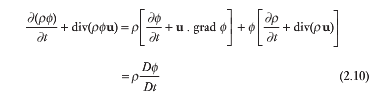的最后一项是不是应该为$D(\phi\rho)/Dt$

第二个公式是
http://openfoam.org/release/2-2-0/numerics-boundedness/
中的第二个公式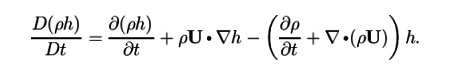左边项应该为$\rho Dh/Dt$,右边第二项应该是div(\rho U h)

• 第一个截图中的公式第一行最后一个等于0，所以书中是正确的。

openfoam网页中这个公式你需要把他后面的内容全部展开后，就是rho h的物质导数，你先尝试自己展开一下，有问题我再打公式。:sunglasses:

BTW, 不需要@我了，我默认所有的帖子都是开放性讨论而不是针对我的。

• 版主，我尝试对第二个公式进行分解，结果如下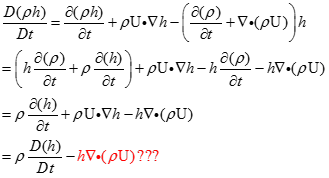另外我注意到，比如说interphasechangeFoam里面的UEqn

    fvVectorMatrix UEqn
(
fvm::ddt(rho, U)
+ fvm::div(rhoPhi, U)
- fvm::Sp(fvc::ddt(rho) + fvc::div(rhoPhi), U)
+ turbulence->divDevRhoReff(rho, U)
);


的第二项，为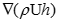而不是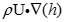，故有此疑问。

• 方程实际要求的是：
\begin{equation}
\frac{\partial \rho\mathbf{U}}{\partial t}+\nabla\cdot(\rho\mathbf{U}\mathbf{U})=0
\end{equation}
对应：

fvVectorMatrix UEqn
(
fvm::ddt(rho, U)
+ fvm::div(rhoPhi, U)


连续性方程：
\begin{equation}
\frac{\partial \rho}{\partial t}+\nabla\cdot(\rho\mathbf{U})=0
\end{equation}
对应：

- fvm::Sp(fvc::ddt(rho) + fvc::div(rhoPhi), U)


因此实际求解的就是：

fvVectorMatrix UEqn
(
fvm::ddt(rho, U)
+ fvm::div(rhoPhi, U)
+ turbulence->divDevRhoReff(rho, U)
);


- fvm::Sp(fvc::ddt(rho) + fvc::div(rhoPhi), U)包含进来的原因为这有利于使求解变量有界以更好地收敛。

CFD中文网 2016 - 2020 | 京ICP备15017992号-2